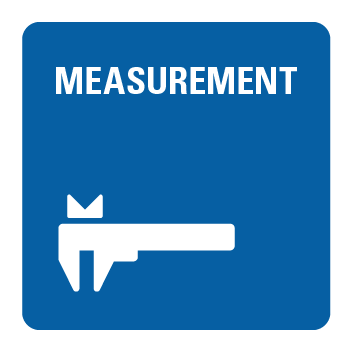Latest Articles
cancel
Showing results for
Did you mean:

## CLAD2017 - DAQmx - Configuration Series 2 of 5SercoSteveBActive Participant

Which of the following are possible contents of Numeric Array Out following execution of the VI?GladysToMeetYou
Member

A

The number of samples per channel terminal on the DAQmx Read function is unwired (default value of -1), so LabVIEW decides how many samples to read based on if samples are acquired continuously or if only a finite number are being acquired. In this case, we are trying to acquire a finite number of samples per channel (3) at a rate of 100 samples per channel per second.

Per LabVIEW Help for the DAQmx Read function:

"If the task acquires a finite number of samples and you set this input to -1, the VI waits for the task to acquire all requested samples, then reads those samples."

So only the 3 samples are read and appear in the 2D Array Output. Also, note that each row corresponds to a channel in the task. Each column corresponds to a sample in that channel.Vinay_Kumar_M_S
Member

B

The Read Function Reads 3 samples per channel. So 2D array with 3 samples in each row will be the output.sankar06
Member

B

Happy to Wirenik35324
Member

BKnight of NI

BThere are only two ways to tell somebody thanks: Kudos and Marked Solutions
Unofficial Forum Rules and Guidelines

b)GladysToMeetYou
Member

Yup, my mistake. Should be B.

Didn't catch that there are 2 channels (Dev1/ai0:1)Ellie90
Member

bMatt-A.
Member

B.qubirt
Member

Bravitejashivuni
Member

A

RAVITEJA STsjabrantes
Member

Hello everyone!DAQmx - Configuration Series 2 of 5

Acquiring 3 samples and reading an 2D array then letter B is the correct answer.

Tarciso Junior
+55 (11) 996-282-103
Skype: Tarciso.junior2
Email: Tsjabrantes@gmail.comSercoSteveBActive Participant

DAQmx Read returns a 2D array of samples. Each row corresponds to a channel in the task. Each column corresponds to a line in that channel.PakAsia
Member

SercoSteveB wrote

Which of the following are possible contents of Numeric Array Out following execution of the VI?DAQmx Read returns a 2D array of samples. Each row corresponds to a channel in the task. Each column corresponds to a line in that channel.

The answer is 2 rows and 3 columns its understandable but what if finite samples is changed to continuous samples selection and number of samples per channel for DAQmx read has value wired in. Please take a look at attached images from CLAD sample exam 2017. If someone can help to understand how to calculate the resulting array of DAQ.

ThankstimmySercoSteveBActive Participant

Hi Timmy

HINT:  The elapsed time returned by Elapsed Time the first time it is called is 0.  Then you will need to work out how quickly each loop will perform the data acquisition.  This should help you with Q4 and Q5.

As for Q6, the structure of the question is confusing me.  If the number of samples per channel value gets to over 100 then you will exceed the default timeout for the DAQmx Read which is 10 seconds.  That only leaves answer A (10) but the fact that NI have used parallel data acquisition suggests to me there is something I have missed.

StevePakAsia
Member

Elapsed Time function do return 0 by first time but I am unable to figure out how to calculate the loop time or what is the relation between rate and number of samples per channel. As per my understanding rate defines the sampling rate and number of samples define how much samples to read from a channel (and allocates memory).

The answer for Q4 is 2rows and 5columns, Q5 is 4rows and 20000columns and answer for Q6 is 10. I am confused here thinking that 1channel=1row but here 1channel is written on multiple rows. I was not able to find a good source of study material to clear things up so that I can calculate the results without using Labview because in the CLAD test software is not available.

Anything with little more clarifications would be appreciated.

timmySercoSteveBActive Participant

You are correct, the acquisition time is defined by the number of samples divided by the sample rate.  For output data you get an array with one row for each channel and a column for each sample taken for that channel.

For Q4:  1 channel is being sampled 5 times, so for after the first iteration of the loop you have an array with 1 row (channel) and 5 columns (samples).  From that point on every additional iteration of loop adds an additional row (with 5 columns) to the array, this is because of the auto-indexing terminal on the While Loop.   The loop iterates twice because the While Loop Stop Condition (elapsed time > 4) is satisfied after the second iteration (when elapsed time = 5).  2 Iterations of the loop gives us a 2D array with 2 rows and 5 columns.

For Q5:  Exactly the same principal as in Q4 but the acquisition time is now 2 seconds (20000 samples divided by 10000 samples/sec).  The Elapsed Time values for each iteration of the loop will be 0, 2, 4, 6.  Only when elapsed time = 6 will the While Loop Stop condition be satisfied.  4 Iterations of the loop gives us a 2D array with 4 rows and 20000 columns.

Hope this helps

StevePakAsia
Member

Thank you Steve for clearing everything. The working functionality of the Elapsed time function and the number of samples divided by the sample rate calculation of the loop timing makes things clear.

timmyA.Bernau
Member

B or C

I have to remember "Each row corresponds to a channel in the task. Each column corresponds to a line in that channel." (by Steve)
So finally B